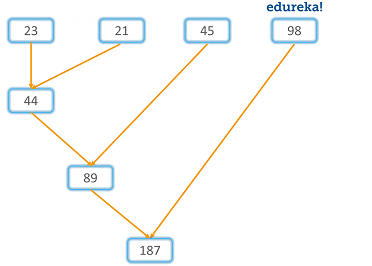# 使用 Python 映射，过滤和缩减函数：所有您需要知道的Python 提供了许多预定义的内置函数，最终用户可以通过调用它们来使用它们。这些功能不仅简化了程序员的工作，而且创建了标准的编码环境。在本文中，您将学习 Python 中的三个令人印象深刻的函数，即 map（），filter 和 reduce（）。

• 什么是 Python 中的 map，filter 和 reduce 函数？

• 在以下范围内使用用户定义的函数和 lambda 函数：

map（）函数

filter（）函数

reduce（）函数

• 一起使用 map（），filter（）和 reduce（）函数

map（）中的 filter（）

filter（）中的 map（）

map（）和 filter（）中带有 reduce（）

## 什么是 Python 中的 map（），filter（）和 reduce（）函数？

map（）函数：

map（）函数是一种高阶函数。如前所述，此函数将另一个函数与一个可迭代序列一起作为参数，并在将该函数应用于序列中存在的每个可迭代序列之后返回输出。其语法如下：

## 在以下范围内使用用户定义的函数和 Lambda 函数：

### map（）中的用户定义函数：

map（）函数可以将用户定义的函数作为参数。这些功能的参数由用户或程序员专门设置。例如：

def newfunc(a):    return a*ax = map(newfunc, (1,2,3,4))  #x is the map objectprint(x)print(set(x))

<位于0x00000284B9AEADD8的地图对象>{16, 1, 4, 9}

def newfunc(a):    return a*ax = map(newfunc, (1,2,3,4))  #x is the map objectprint(x)print(list(x))

<位于0x00000284B9AEA940的地图对象>[1, 4, 9, 16]

def func(a, b):    return a + b a = map(func, [2, 4, 5], [1,2,3])print(a)print(tuple(a))

<位于0x00000284B9BA1E80的地图对象>(3, 6, 8)

### map（）中的 Lambda 函数：

Lambda 函数是具有任何名称的函数。这些功能通常作为参数提供给其他功能。现在让我们尝试将 lambda 函数嵌入 map（）函数中。考虑以下示例：

tup= (5, 7, 22, 97, 54, 62, 77, 23, 73, 61)newtuple = tuple(map(lambda x: x+3 , tup)) print(newtuple)

(8, 10, 25, 100, 57, 65, 80, 26, 76, 64)

## filter（）函数：

filter（）函数用于创建由值组成的输出列表，该值针对该值返回 true。它的语法如下：

def func(x):    if x>=3:        return xy = filter(func, (1,2,3,4))  print(y)print(list(y))

<位于0x00000284B9BBCC50的过滤器对象>[3, 4]

### 在 filter（）中使用 lambda：

y = filter(lambda x: (x>=3), (1,2,3,4))print(list(y))

[3，4]

### reduce（）函数：from functools import reducereduce(lambda a,b: a+b,[23,21,45,98])

Python 中的 map（），filter（）和 reduce（）函数可以一起使用。

## 一起使用 map（），filter（）和 reduce（）函数：

### 在 map（）中使用 filter（）：

c = map(lambda x:x+x,filter(lambda x: (x>=3), (1,2,3,4)))print(list(c))

### 在 filter（）中使用 map（）：

c = filter(lambda x: (x>=3),map(lambda x:x+x, (1,2,3,4)))  #lambda x: (x>=3)print(list(c))

### 在 reduce（）中使用 map（）和 filter（）：

d = reduce(lambda x,y: x+y,map(lambda x:x+x,filter(lambda x: (x>=3), (1,2,3,4)))) print(d)## 评论